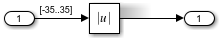## Derived Ranges in Design Error Detection

When you specify minimum and maximum values for a signal or data in a model, these values define a design range.

During design error detection, the software analyzes the model behavior and computes the values that can occur during simulation for:

• Block Outports

• Stateflow® local data

The range of these values is called a derived range.

The Use specified input minimum and maximum values parameter in the Configuration Parameters dialog box, on the Design Verifier pane, if enabled, tells the analysis to consider the design ranges on the model input ports as constraints when calculating the derived ranges. By default, the Use specified input minimum and maximum values parameter is enabled.

If Use specified input minimum and maximum values is disabled, the software does not restrict the signals when computing the derived ranges.

To see how this process works, consider the following model.In this model, the design ranges are:

• Inport block: [–35..35]

• Abs block output: [0..30]

Given the design range on the Inport block, the only possible values for the Abs block output are values from 0 to 35. Therefore, the derived range for the Abs block is [0..35].

However, if you disable the Use specified input minimum and maximum values parameter, the analysis calculates the derived ranges based on unrestricted values of the input ports of the model. In the preceding model, the only valid outputs of the Abs block are nonnegative numbers. Consequently, the derived range for the Abs block is [0..Inf].